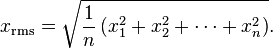## Root Mean Square(RMS) Calculator

 Enter Inputs 5,20,40,80,100 Enter the Numbers with Comma separated(,)
 Result: No.of Inputs Root Mean Square

The Root Mean Square Calculator to calculate the Root Mean Square(RMS) or Quadratic Mean for set of varying data.

### Root Mean Square formula

In the case of a set of n values {x1, x2,x3, ... xn}, Then RMS:For example, a set of n values {5,20,40,80,100}, then Root Mean Square = 60.7042.

Thinkcalculator.com provides you helpful and handy calculator resources.